# Binomial distribution. The probability density function

The following calculator is made for the binomial distribution, the calculation of the probability density function.
What is the binomial distribution? This is the probability of occurrence of a certain event in repeated and mutually independent tests.
If each new test probability of occurrence of an event is p (range [0, 1]), the number of occurrences of the event Y with n independent trials is a random variable that takes the value k = 1, 2, .., n with the following probabilities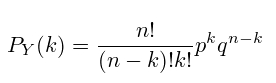, where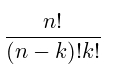- this is the binomial coefficient, and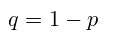The expectation of the value of having a binomial distribution will be considered: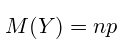and the variance, respectively: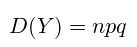The following online calculator calculates P (k) and build a distribution schedule for you specified p and n.

﻿

Binomial distribution
Function density probability:
Mathematical waiting:
Dispersion: